## Friday, October 17, 2014

### federgraph-special-sample

The last blog entry was about a special case. Today I am blogging about the special sample, which contains the special case. In the setup of the sample I can change parameter L - the length of all the springs - over the whole interesting range.

### Sample Situations

I have identified a number of situations/cases and uploaded two scilab source files to pastebin. It is special-sample-2D with a 2D graph, and special-sample-3D with a 3D plot. These files contain more info about the concrete setup, and can be used to create independent graphical output in scilab.

a) L = -a/2 = -50 (all springs under tension)
b) L = 0 (force cone, Kraftkegel)
c) L = a/2 = 50 (single equilibrium at centroid)
d) L = ro = 57 (circles intersect at centroid)
e) L = w3/(1+w3) = 63 (touch down at corner C)
f) L = 83 (bifurcation)
g) L = h = 86 (special case one, equilibrium at midpoint of side)
h) L = a = 100 (no vertical edge/jump)
i) L = 115 (centroid dome)
j) L = w3/(w3-1) = 237 (delta peak take off)

### Screenshot Series

It is difficult to describe in words. Therefore the screenshots are the meat of this blog post - to be used them as a starting point for further exploration. I have included screenshots for intermediate situations in between the special points listed above.

Screenshot 1) L = -50 = negative jump
Screenshot 2) L = 0 = force cone
Screenshot 3) L = 50 = a/2 = circles intersect at mid of triangle side
Screenshot 4) L = 57 = ro = circles intersect at centroid
Screenshot 5) L = 63 = w3/(1+w3) = touch down at point C
Screenshot 6) L = 74
Screenshot 7) L = 83 = touch down of curve
Screenshot 8) L = 86 = h = w3/2 * a = circles touch side
Screenshot 9) L = 100 = a = no jump, only a slope
Screenshot 10) L = 115
Screenshot 11) L = 120
Screenshot 12) L = 140
Screenshot 13) L = 170
Screenshot 14) L = 190
Screenshot 15) L = 210
Screenshot 16) L = 237 = w3/(1-w3) slope vanishes and peak touches floor in point C
Screenshot 17) L = 237 = same as 16, but different perspective
Screenshot 18) L = 300 = delta no longer touches plane
Screenshot 19) L = 490 = curve bends

### Screenshot 1 (L = -50)

This is the only screenshot for a negative value of L. Notice the direction of the jump. The 3D graph looks like a top down view, but notice that it is actually viewed bottom up. The 3D surface represents the absolute value of the force, which stretches from possibly zero (around the origin) to positive values everywhere far from the origin, as the 2D graph on the right hand side shows. I decided to let the triangle point to the right. Otherwise the 2D graph would appear rotated 90 degrees, which is the normal case, where the triangle points upwards. Note that the gray circles for the actual length of the springs touch each other. The peak of the yellow curve points down to the centroid of the triangle.Screenshot 1

### Screenshot 2 (L = 0)

This is indeed a special case.The force surface plot is a cone. There is no problem when you move point P over a corner. We only see one equilibrium at the centroid. The force in any of the springs will be positive - tension, not pressure.Screenshot 2

### Screenshot 3 (L = 50)

The circles touch at the midpoint of a side. This is the same as in Screenshot 1, but the spring will be under pressure when point P is moved towards the corner where it is attached. We see a vertical edge exactly where the corners of the triangle are. This is where the direction of the force will turn around. There is still just one equilibrium - where the surface touches plane z = 0.Screenshot 3

### Screenshot 4 (L = 57 = ro)

This is special only because the circles intersect at the centroid.Screenshot 4

### Screenshot 5 (L = 63)

Notice that in this situation the peak will touch the plane at point C - the triangle corner (x3, y3) - which is on the x-axis in this special sample.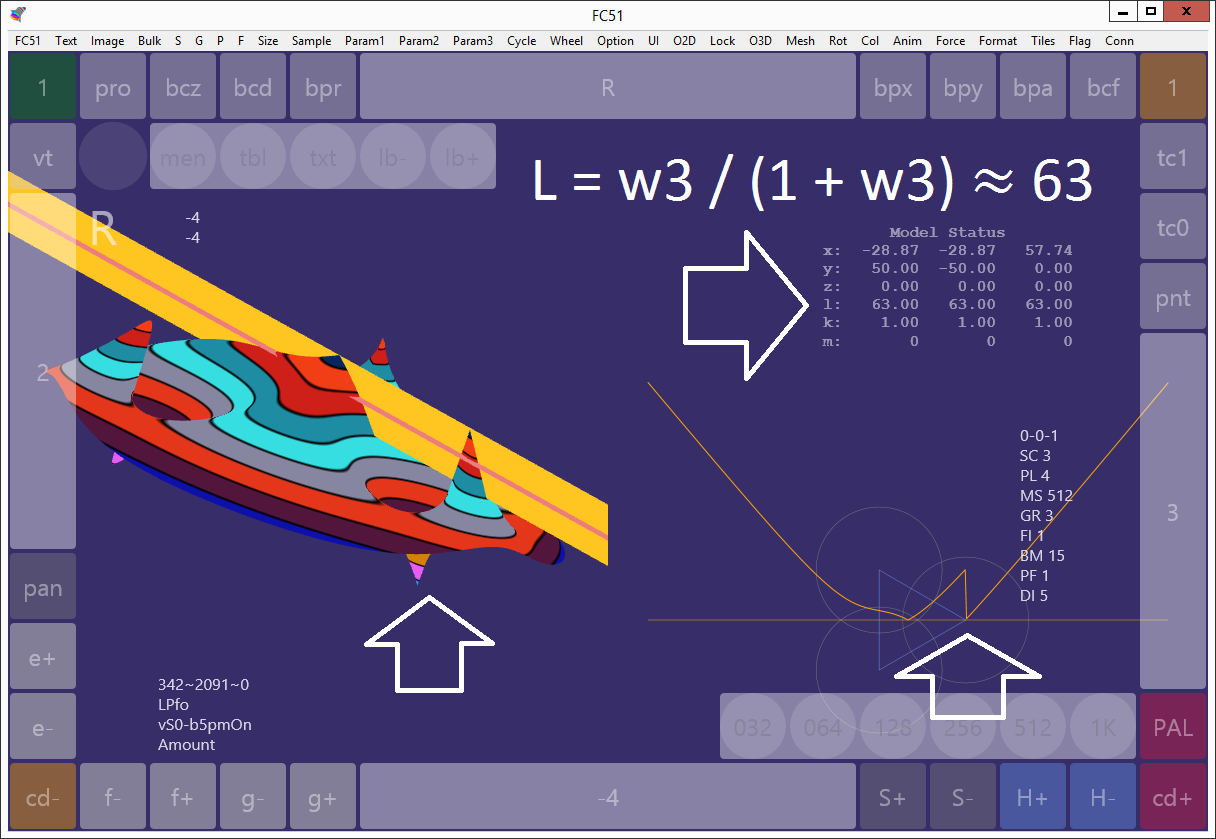Screenshot 5

### Screenshot 6 (L = 74)

Notice the slope which is not present in the previous picture. It has appeared, so I thought it is worth a screenshot.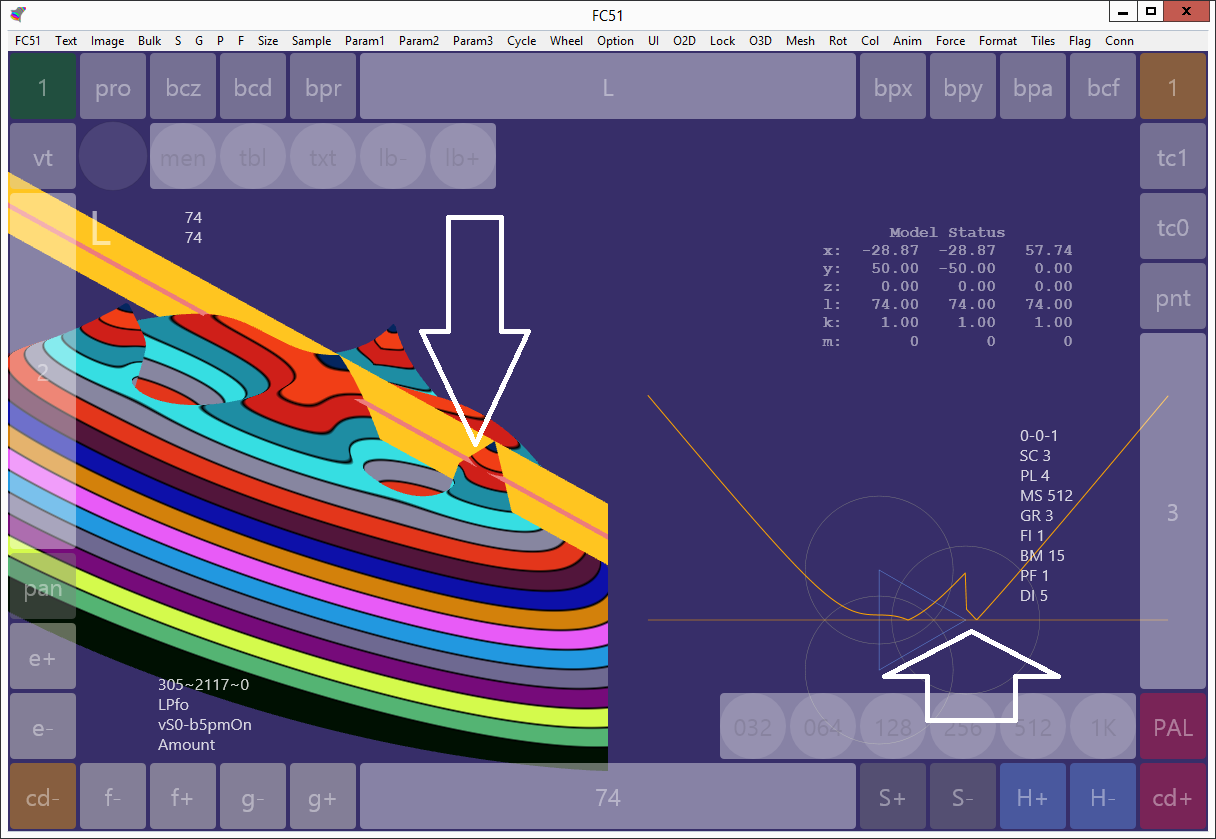Screenshot 6

### Screenshot 7 (L = 83)

The slope is getting bigger, but I want to turn your attention to the fact that the curve has touched the plane. We now have three zeros within the plotted section. Overall we have even more. Until now I have not yet determined the expression for the exact value of L when this happens.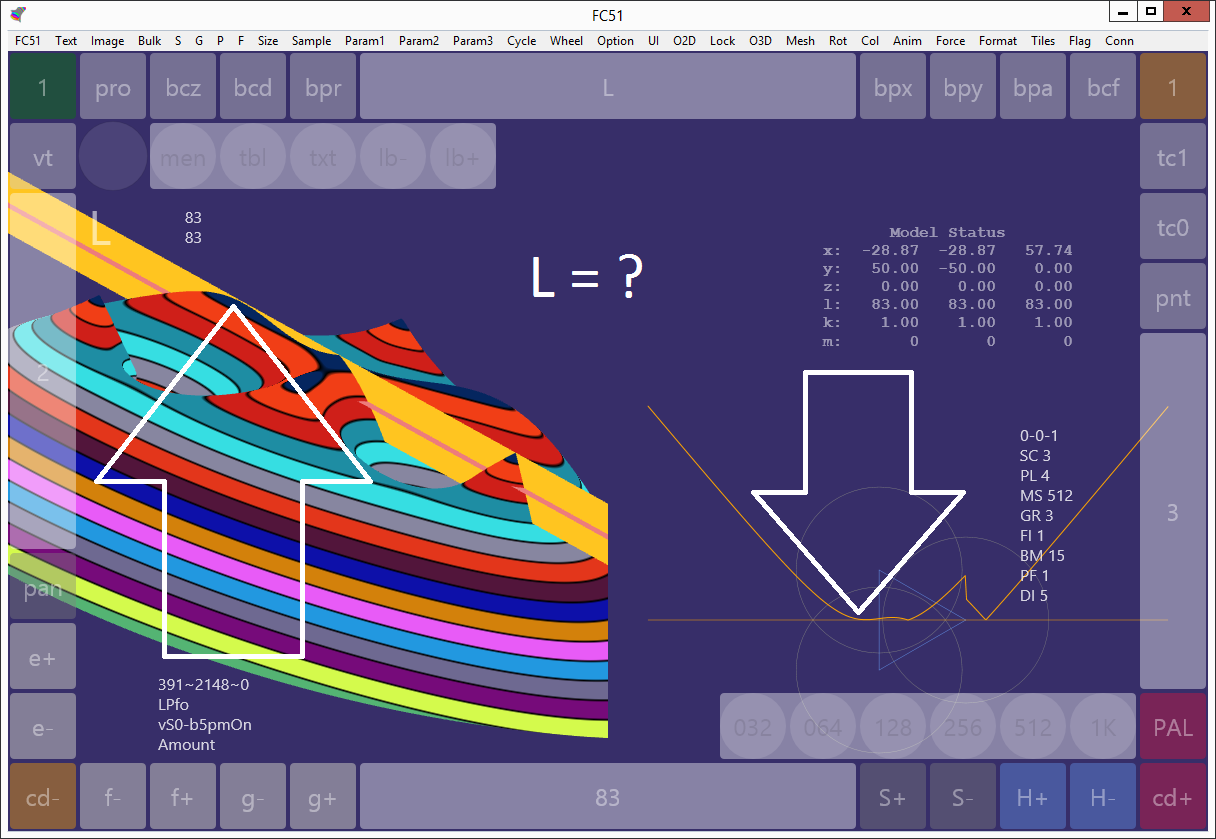Screenshot 7

### Screenshot 8 (L = h = 86 = w3/2 * a)

This is the special case from the last blog post. The curve is touching the plane exactly at the middle of the triangle side.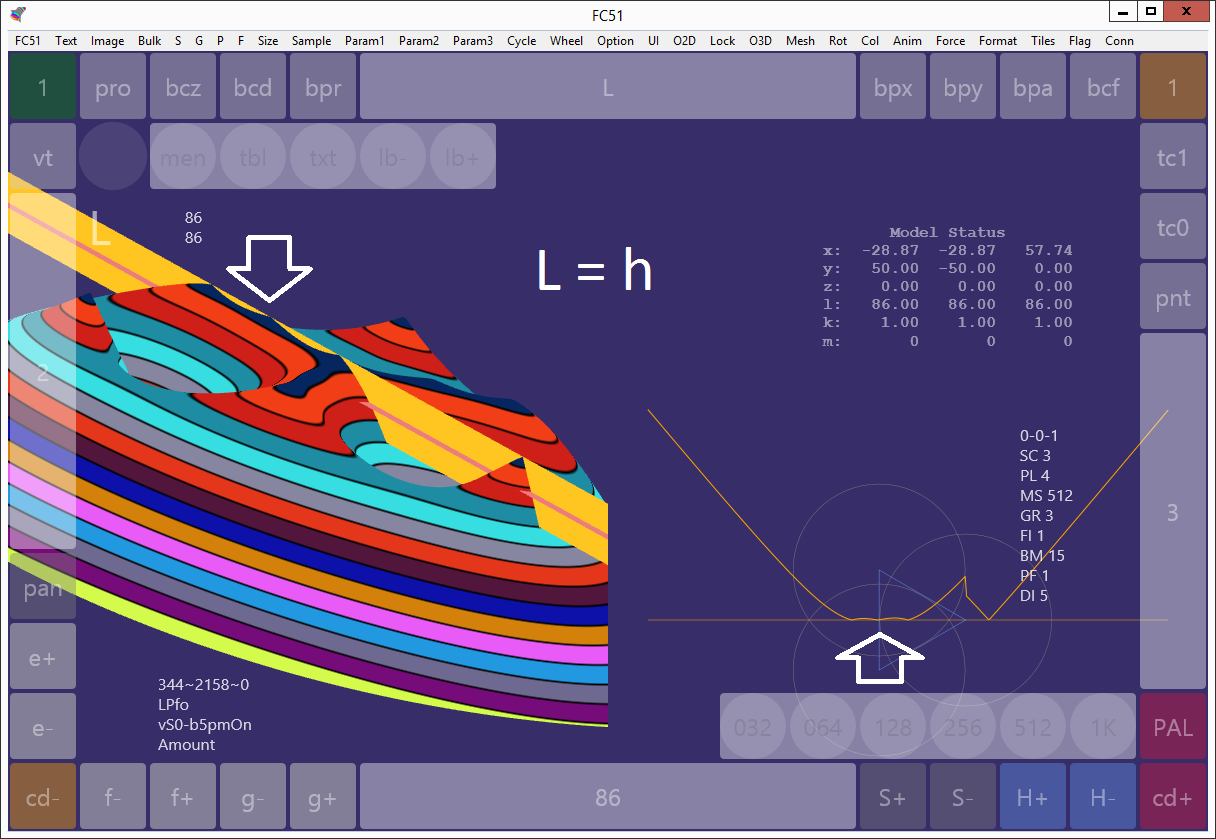Screenshot 8

### Screenshot 9 (L = 100 = a)

This situation is special because the jump (vertical edge) temporarily disappeared. Also note that the one spot - that was situated on the triangle side in the last screenshot - is now moving closer to the centroid.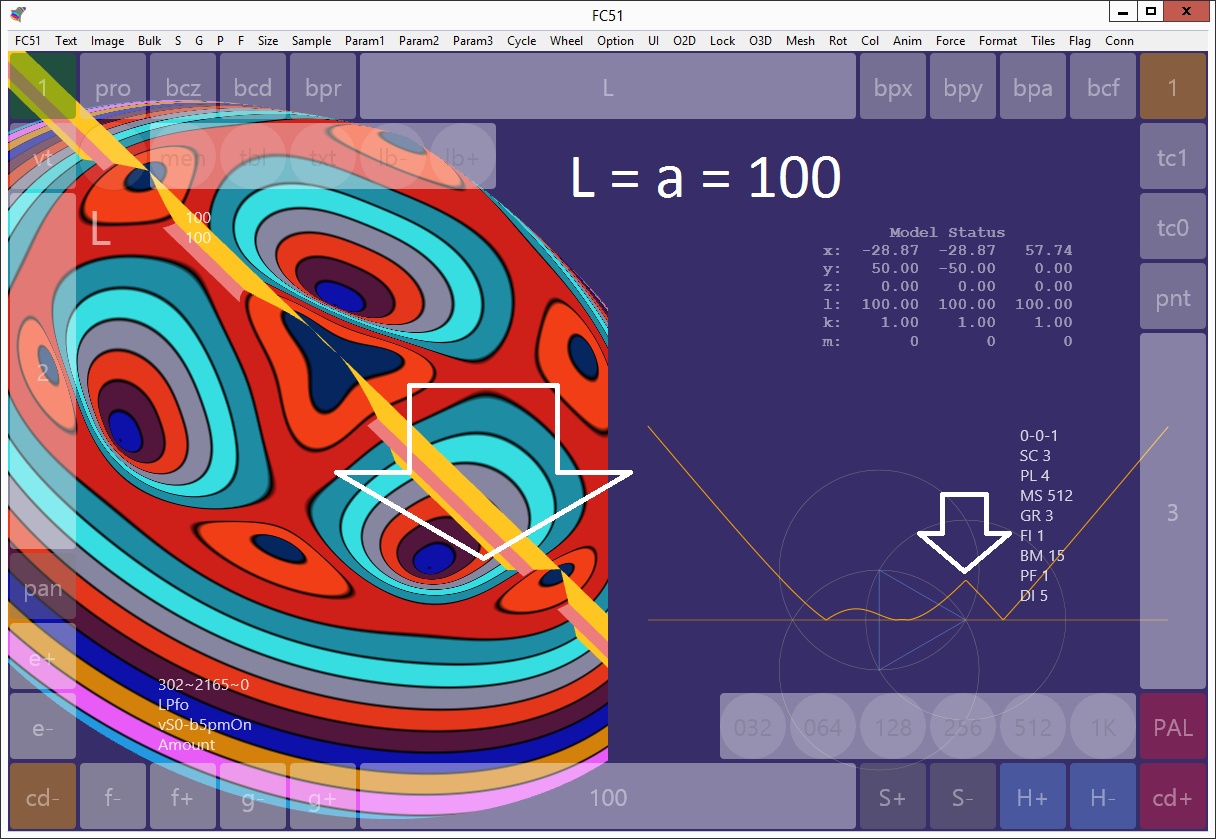Screenshot 9

### Screenshot 10 (L = 115)

Close to the centroid the surface smoothly touches the plane and the shape of the surface in that area is almost circular. I still have to find a name for this situation. Also note the six-corner-shape.Screenshot 10

### Screenshot 11 (L = 120)

What seemed to be round in the middle is now looking like a triangle.Screenshot 11

### Screenshot 12 (L = 140)

The mold has appeared again. The shape in the center is looking like a delta. Notice the slope which leads to point C.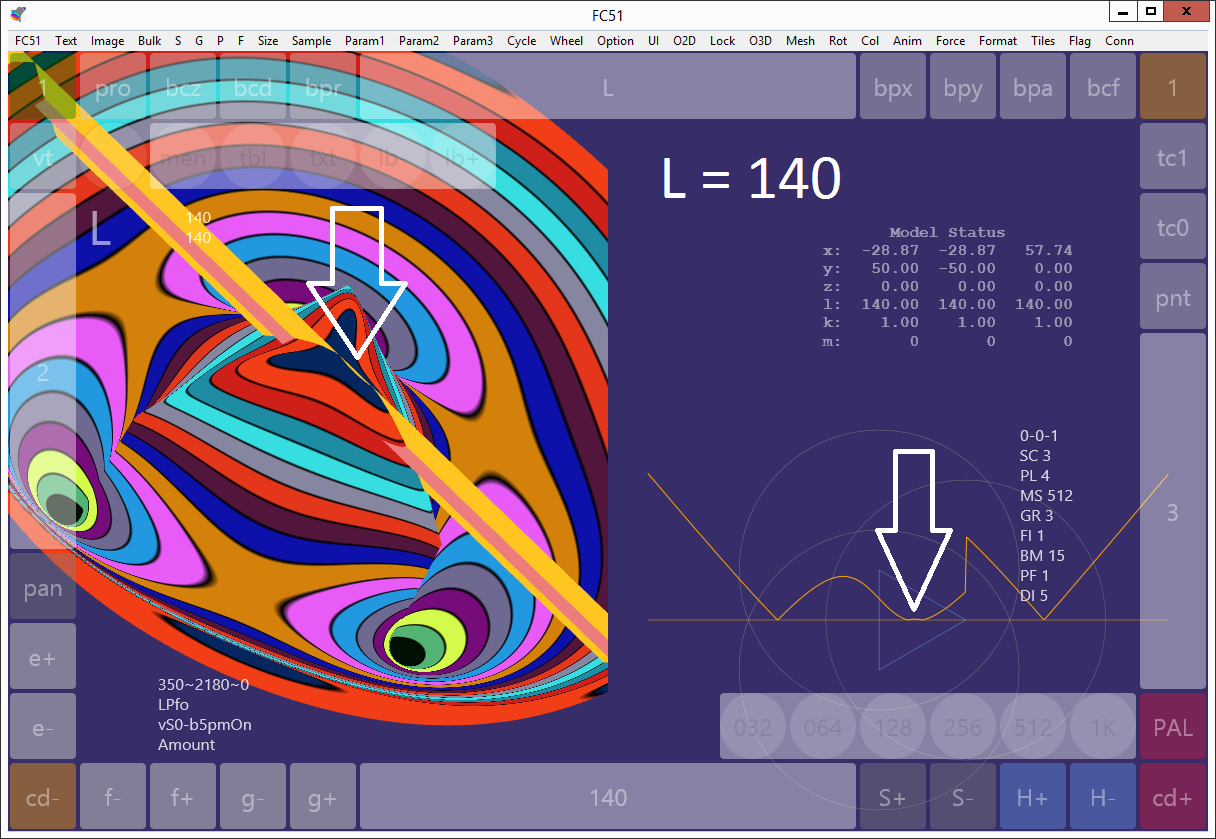Screenshot 12

### Screenshot 13 (L = 170)

The delta is taking shape. The slope is getting shorter.Screenshot 13

### Screenshot 14 (L = 190)

For this screenshot I have adjusted the texture parameters - the width and offset of the colored rings. My interest is now focused on the slope.Screenshot 14

### Screenshot 15 (L = 210)

The slope has become very short here. It is about to disappear. This is best seen from the side - so I changed rotation (and zoom).Screenshot 15

### Screenshot 16 (L = 237 = w3/(1-w3) )

Now the slope is gone, and the peak touches down to the plane exactly at point C.Screenshot 16

### Screenshot 17 (L = 237)

This is the same situation as in screenshot 16, but with different view parameters.Screenshot 17

### Screenshot 18 (L = 300)

Notice that the peak no longer touches the plane. Since I increased the plotted range you can see the rim. The six equilibria around the rim are almost sinking into it.Screenshot 18

### Screenshot 19 (L = 490)

Parameter L is now pretty much out of bounds. Notice how the outer slope appears curved.Screenshot 19

Now imagine how everything will fit if we translate part of the curve, mirror another part of the curve, and how that would simplify the equations.